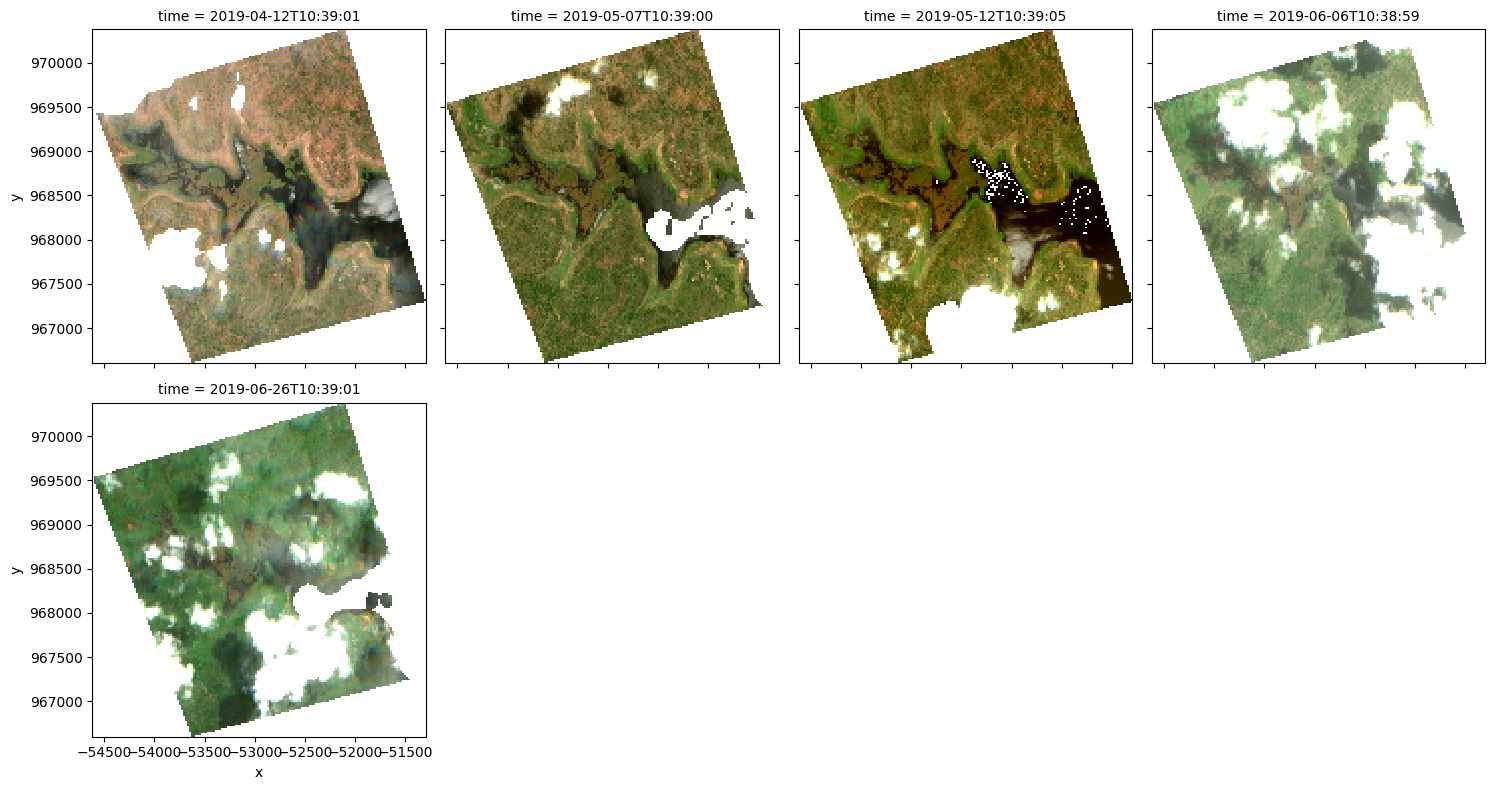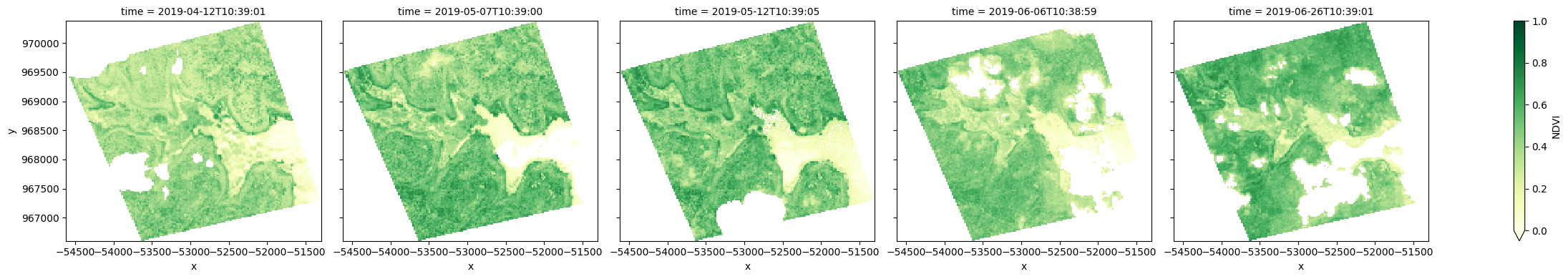# Run analyses on multiple polygons¶

Keywords: spatial analysis; polygons, data used; sentinel-2, python package; GeoPandas, band index; NDVI

## Background¶

Many users need to run analyses on their own areas of interest. A common use case involves running the same analysis across multiple polygons in a vector file (e.g. ESRI Shapefile or GeoJSON). This notebook will demonstrate how to use a vector file and the Open Data Cube to extract satellite data from Digital Earth Africa to match individual polygon geometries.

## Description¶

If we have a vector file containing multiple polygons, we can use the python package geopandas to open it as a GeoDataFrame. We can then iterate through each geometry and extract satellite data corresponding with the extent of each geometry. Further anlaysis can then be conducted on each resulting xarray.Dataset.

In this notebook, we demonstrate how to retrieve data for each polygon and perform an analysis. The example analysis in this notebook is to load and plot the normalised difference vegetation index (NDVI). This is conducted through the following steps:

1. Open the file of polygons using geopandas.

2. Iterate through the generated GeoDataFrame, extracting satellite data from DE Africa’s Open Data Cube.

3. Calculate NDVI as an example analysis on one of the extracted satellite timeseries.

4. Plot NDVI for the polygon extent.

## Getting started¶

To run this analysis, run all the cells in the notebook, starting with the “Load packages” cell.

Please note the use of datacube.utils package geometry: this is important for saving the coordinate reference system of the incoming shapefile in a format that the Digital Earth Africa query can understand.

:

%matplotlib inline

import datacube
import matplotlib.pyplot as plt
import geopandas as gpd
from datacube.utils import geometry

from deafrica_tools.bandindices import calculate_indices
from deafrica_tools.plotting import rgb, map_shapefile
from deafrica_tools.spatial import xr_rasterize
from deafrica_tools.classification import HiddenPrints


### Connect to the datacube¶

Connect to the datacube database to enable loading Digital Earth Australia data.

:

dc = datacube.Datacube(app='Analyse_multiple_polygons')


## Analysis parameters¶

• time_range : Enter a time range for your query, e.g.('2019-01', '2019-12') if you wanted data from all of 2019

• vector_file : A path to a vector file (ESRI Shapefile or GeoJSON) containing polygons to load. For this example we have provided a demonstration Shapefile

• attribute_col : A column in the vector file used to label the output xarray datasets containing satellite images. Each row of this column should have a unique identifier

• products : A list of product names to load from the datacube, e.g. ['ls8_c2l2', 'ls7_c2l2']. In this example we will use only Sentinel-2 data, ['s2_l2a']

• measurements : A list of band names to load from the satellite product, e.g. ['red', 'green']

• resolution : The spatial resolution of the loaded satellite data in the x and y directions in metres. For this Sentinel-2 example, we have selected (-20, 20)

• output_crs : The coordinate reference system/map projection to load data into, e.g. 'EPSG:6933' to load data in an Equal Area projection for Africa

:

time_range = ('2019-04', '2019-07')

vector_file = '../Supplementary_data/Analyse_multiple_polygons/multiple_polygons.shp'
attribute_col = 'id'

products = ['s2_l2a']
measurements = ['red', 'green', 'blue', 'nir']
resolution = (-20, 20)
output_crs = 'EPSG:6933'


### Look at the structure of the vector file¶

Import the file and take a look at how the file is structured so we understand what we are iterating through. There are three polygons in the file:

We will also update the id column to give each polygon a unique identifier. This will be used to identify the satelllite data corresponding with the polygon in the results dictionary

:

#read shapefile

gdf['id']=range(0, len(gdf))

#print gdf

:

id class geometry
0 0 0 POLYGON ((-0.55603 7.66642, -0.52764 7.67401, ...
1 1 0 POLYGON ((-0.53018 7.64633, -0.49693 7.65515, ...
2 2 0 POLYGON ((-0.56600 7.62164, -0.54007 7.62830, ...

We can then plot the geopandas.GeoDataFrame using the function map_shapefile to make sure it covers the area of interest we are concerned with:

:

map_shapefile(gdf, attribute=attribute_col)


### Create a datacube query object¶

We then create a dictionary that will contain the parameters that will be used to load data from the Digital Earth Africa datacube:

Note: We do not include the usual x and y spatial query parameters here, as these will be taken directly from each of our vector polygon objects.

:

query = {'time': time_range,
'measurements': measurements,
'resolution': resolution,
'output_crs': output_crs,
}

query

:

{'time': ('2019-04', '2019-07'),
'measurements': ['red', 'green', 'blue', 'nir'],
'resolution': (-20, 20),
'output_crs': 'EPSG:6933'}


Here we will iterate through each row of the geopandas.GeoDataFrame and load satellite data. The results will be appended to a dictionary object which we can later index to analyse each dataset.

:

# Dictionary to save results
results = {}

# A progress indicator
i = 0

# Loop through polygons in geodataframe and extract satellite data
for index, row in gdf.iterrows():

print(" Feature {:02}/{:02}\r".format(i + 1, len(gdf)),
end='')

# Get the geometry
geom = geometry.Geometry(row.geometry.__geo_interface__,
geometry.CRS(f'EPSG:{gdf.crs.to_epsg()}'))

# Update dc query with geometry
query.update({'geopolygon': geom})

# Load landsat (hide print statements)
with HiddenPrints():
products=products,
min_gooddata=0.75,
group_by='solar_day',
**query)

# Generate a polygon mask to keep only data within the polygon
with HiddenPrints():

# Mask dataset to set pixels outside the polygon to NaN

# Append results to a dictionary using the attribute
# column as an key
results.update({str(row[attribute_col]) : ds})

# Update counter
i += 1

 Feature 03/03


## Further analysis¶

Our results dictionary will contain xarray objects labelled by the unique attribute_col values we specified in the Analysis parameters section:

:

results

:

{'0': <xarray.Dataset>
Dimensions:      (time: 5, y: 192, x: 178)
Coordinates:
* time         (time) datetime64[ns] 2019-04-17T10:50:37 ... 2019-06-26T10:...
* y            (y) float64 9.762e+05 9.761e+05 ... 9.724e+05 9.723e+05
* x            (x) float64 -5.365e+04 -5.363e+04 ... -5.013e+04 -5.011e+04
spatial_ref  int32 6933
Data variables:
red          (time, y, x) float32 nan nan nan nan nan ... nan nan nan nan
green        (time, y, x) float32 nan nan nan nan nan ... nan nan nan nan
blue         (time, y, x) float32 nan nan nan nan nan ... nan nan nan nan
nir          (time, y, x) float32 nan nan nan nan nan ... nan nan nan nan
Attributes:
crs:           EPSG:6933
grid_mapping:  spatial_ref,
'1': <xarray.Dataset>
Dimensions:      (time: 7, y: 169, x: 178)
Coordinates:
* time         (time) datetime64[ns] 2019-04-17T10:50:37 ... 2019-07-06T10:...
* y            (y) float64 9.738e+05 9.738e+05 ... 9.704e+05 9.704e+05
* x            (x) float64 -5.115e+04 -5.113e+04 ... -4.763e+04 -4.761e+04
spatial_ref  int32 6933
Data variables:
red          (time, y, x) float32 nan nan nan nan nan ... nan nan nan nan
green        (time, y, x) float32 nan nan nan nan nan ... nan nan nan nan
blue         (time, y, x) float32 nan nan nan nan nan ... nan nan nan nan
nir          (time, y, x) float32 nan nan nan nan nan ... nan nan nan nan
Attributes:
crs:           EPSG:6933
grid_mapping:  spatial_ref,
'2': <xarray.Dataset>
Dimensions:      (time: 7, y: 189, x: 166)
Coordinates:
* time         (time) datetime64[ns] 2019-04-12T10:53:19 ... 2019-06-26T10:...
* y            (y) float64 9.704e+05 9.704e+05 ... 9.666e+05 9.666e+05
* x            (x) float64 -5.461e+04 -5.459e+04 ... -5.133e+04 -5.131e+04
spatial_ref  int32 6933
Data variables:
red          (time, y, x) float32 nan nan nan nan nan ... nan nan nan nan
green        (time, y, x) float32 nan nan nan nan nan ... nan nan nan nan
blue         (time, y, x) float32 nan nan nan nan nan ... nan nan nan nan
nir          (time, y, x) float32 nan nan nan nan nan ... nan nan nan nan
Attributes:
crs:           EPSG:6933
grid_mapping:  spatial_ref}


Enter one of those values below to index our dictionary and conduct further analsyis on the satellite timeseries for that polygon.

:

key = '2'


### Plot an RGB image¶

We can now use the dea_plotting.rgb function to plot our loaded data as a three-band RGB plot:

:

rgb(results[key], col='time', size=4)### Calculate NDVI and plot¶

We can also apply analyses to data loaded for each of our polygons. For example, we can calculate the normalised difference vegetation index (NDVI) to identify areas of growing vegetation.

Note: NDVI can be calculated using the calculate_indices function, imported from deafrica_tools.bandindices. Here, we use satellite_mission='s2' since we are working with Sentinel-2 data.

:

# Calculate band index
ndvi = calculate_indices(results[key], index='NDVI', satellite_mission='s2')

# Plot NDVI for each polygon for the time query
ndvi.NDVI.plot(col='time', cmap='YlGn', vmin=0.0, vmax=1, figsize=(25, 4))
plt.show()Contact: If you need assistance, please post a question on the Open Data Cube Slack channel or on the GIS Stack Exchange using the open-data-cube tag (you can view previously asked questions here). If you would like to report an issue with this notebook, you can file one on Github.

Compatible datacube version:

:

print(datacube.__version__)

1.8.6


Last Tested:

:

from datetime import datetime
datetime.today().strftime('%Y-%m-%d')

:

'2022-07-07'